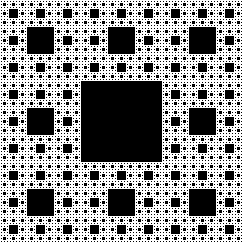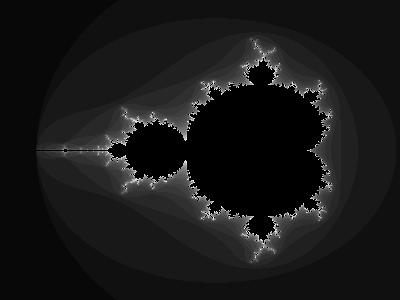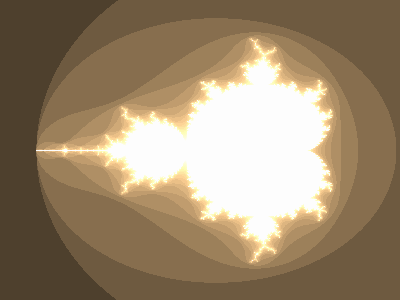## Fractal Rendering in Emacs

Taking advantage of Emacs’ `image-mode` and the handy Netpbm format it’s possible to generate and render images inside Emacs using Elisp. This function will generate a Sierpinski carpet and display the result in a buffer.

``````(defun sierpinski (s)
(pop-to-buffer (get-buffer-create "*sierpinski*"))
(fundamental-mode) (erase-buffer)
(labels ((fill-p (x y)
(cond ((or (zerop x) (zerop y)) "0")
((and (= 1 (mod x 3)) (= 1 (mod y 3))) "1")
(t (fill-p (/ x 3) (/ y 3))))))
(insert (format "P1\n%d %d\n" s s))
(dotimes (y s) (dotimes (x s) (insert (fill-p x y) " "))))
(image-mode))
``````

It’s best called with powers of three,

``````(sierpinski (expt 3 5))
``````This one should look quite familiar. Using the same technique,

``````(defun mandelbrot ()
(pop-to-buffer (get-buffer-create "*mandelbrot*"))
(let ((w 400) (h 300) (d 32))
(fundamental-mode) (erase-buffer)
(set-buffer-multibyte nil)
(insert (format "P6\n%d %d\n255\n" w h))
(dotimes (y h)
(dotimes (x w)
(let* ((cx (* 1.5 (/ (- x (/ w 1.45)) w 0.45)))
(cy (* 1.5 (/ (- y (/ h 2.0)) h 0.5)))
(zr 0) (zi 0)
(v (dotimes (i d d)
(if (> (+ (* zr zr) (* zi zi)) 4) (return i)
(psetq zr (+ (* zr zr) (- (* zi zi)) cx)
zi (+ (* (* zr zi) 2) cy))))))
(insert-char (floor (* 256 (/ v 1.0 d))) 3))))
(image-mode)))
``````Tweak it with a colormap,

``````(defun colormap (v)
"Given a value between 0 and 1.0, insert a P6 color."
(dotimes (i 3)
(insert-char (floor (* 256 (min 0.99 (sqrt (* (- 3 i) v))))) 1)))
``````One of the project ideas on my mental back-burner of things I’ll never get to is to create a little graphics library for Elisp. It would use a technique like this to pull it off. Assuming support was compiled in, Emacs can even render SVGs to a buffer, so creating a rich graphics library wouldn’t be difficult at all. Plus, unlike bare Elisp, it would be fast.

Have a comment on this article? Start a discussion in my public inbox by sending an email to ~skeeto/public-inbox@lists.sr.ht , or see existing discussions.